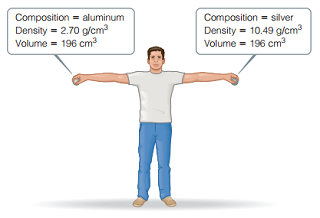# Problem: Consider the two spheres shown here, one made of silver and the other of aluminum.What is the mass of the aluminum sphere in kg?

###### FREE Expert Solution

$\overline{)\mathbf{density}\mathbf{=}\frac{\mathbf{mass}}{\mathbf{volume}}}$

density = 2.70 cm3

volume = 196 cm3

1 kg = 103 g###### Problem Details

Consider the two spheres shown here, one made of silver and the other of aluminum.What is the mass of the aluminum sphere in kg?